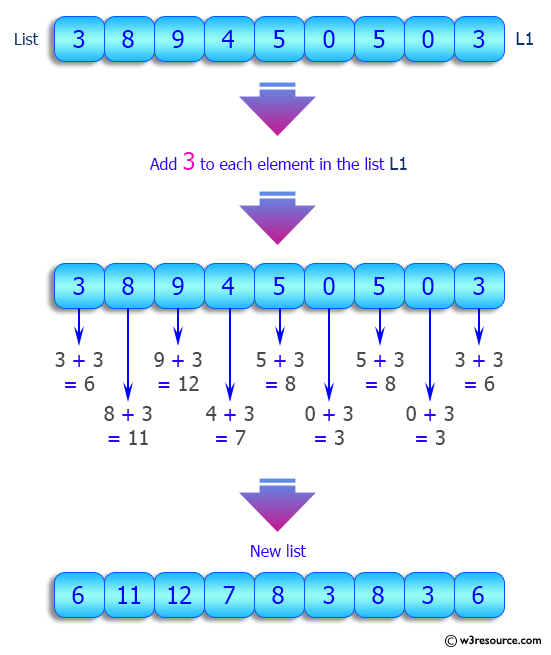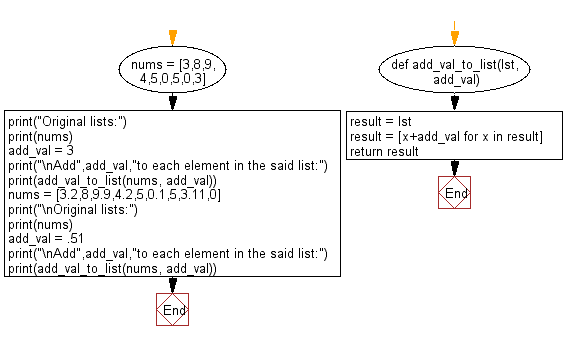﻿ Python: Add a number to each element in a given list of numbers - w3resource# Python: Add a number to each element in a given list of numbers

## Python List: Exercise - 174 with Solution

Write a Python program to add a number to each element in a given list of numbers.

Sample Solution:

Python Code:

``````def add_val_to_list(lst, add_val):
result = lst
result = [x+add_val for x in result]
return result
nums = [3,8,9,4,5,0,5,0,3]
print("Original lists:")
print(nums)
nums = [3.2,8,9.9,4.2,5,0.1,5,3.11,0]
print("\nOriginal lists:")
print(nums)
```
```

Sample Output:

```Original lists:
[3, 8, 9, 4, 5, 0, 5, 0, 3]

Add 3 to each element in the said list:
[6, 11, 12, 7, 8, 3, 8, 3, 6]

Original lists:
[3.2, 8, 9.9, 4.2, 5, 0.1, 5, 3.11, 0]

Add 0.51 to each element in the said list:
[3.71, 8.51, 10.41, 4.71, 5.51, 0.61, 5.51, 3.62, 0.51]
```

Pictorial Presentation:Flowchart:## Visualize Python code execution:

The following tool visualize what the computer is doing step-by-step as it executes the said program:

Python Code Editor:

Have another way to solve this solution? Contribute your code (and comments) through Disqus.

What is the difficulty level of this exercise?

Test your Python skills with w3resource's quiz

﻿

## Python: Tips of the Day

Floor Division:

When we speak of division we normally mean (/) float division operator, this will give a precise result in float format with decimals.

For a rounded integer result there is (//) floor division operator in Python. Floor division will only give integer results that are round numbers.

```print(1000 // 300)
print(1000 / 300)```

Output:

```3
3.3333333333333335```